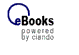Schnellsuche zur Expertensuche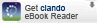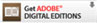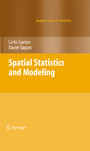Großes Bild

Spatial Statistics and Modeling
von: Carlo Gaetan, Xavier Guyon
Springer-Verlag, 2009
ISBN: 9780387922577

 Format:  PDF geeignet für: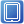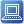Typ: B (paralleler Zugriff)KurzinformationSpatial statistics are useful in subjects as diverse as climatology, ecology, economics, environmental and earth sciences, epidemiology, image analysis and more. This book covers the best-known spatial models for three types of spatial data: geostatistical data  (stationarity, intrinsic models, variograms, spatial regression and space-time models), areal data  (Gibbs-Markov fields and spatial auto-regression) and point pattern data (Poisson, Cox, Gibbs and Markov point processes). The level is relatively advanced, and the presentation concise but complete.  The most important statistical methods and their asymptotic  properties are described, including estimation in geostatistics, autocorrelation and second-order statistics, maximum likelihood methods, approximate inference using the pseudo-likelihood or Monte-Carlo simulations, statistics for point processes and Bayesian hierarchical models. A chapter is devoted to Markov Chain Monte Carlo simulation (Gibbs sampler, Metropolis-Hastings algorithms and exact simulation).A large number of real examples are studied with R, and each chapter ends with a set of theoretical and applied exercises. While a foundation in  probability and mathematical statistics is assumed,  three appendices introduce some necessary background. The book is accessible to senior undergraduate students with a solid math background  and Ph.D. students in statistics. Furthermore, experienced statisticians and researchers in the above-mentioned fields will find the book valuable as a mathematically sound reference. This book is the English translation of Modélisation et Statistique Spatiales published by Springer in the series Mathématiques & Applications, a series established by Société de Mathématiques Appliquées et Industrielles (SMAI).nach obenMehr zum Inhalt
 Kapitelübersicht Kurzinformation Inhaltsverzeichnis Leseprobe Blick ins Buch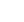Navigation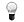Info
© 2008-2020 ciando GmbH | Impressum | Kontakt | F.A.Q. | Datenschutz